9923170071 / 8108094992 info@dimensionless.in
Select Page

Bi-Variate Analysis

In my previous post, we have covered Uni-Variate Analysis as an initial stage of data-exploration process. In this post, we will cover the bi-variate analysis. Objective of the bi-variate analysis is to understand the relationship between each pair of variables using statistics and visualizations. We need to analyze relationship between:

• the target variable and each predictor variable
• two predictor variables

### Why Analyze Relationship between Target Variable and each Predictor Variable

It is important to analyze the relationship between the predictor and the target variables to understand the trend for the following reasons:

• The bi-variate analysis and our model should communicate the same story. This will help in understand and analysing the accuracy of our models, and to make sure, that our model has not over-fit the training data.
• If the data has too many predictor variables, we should include only those predictor variables in our regression models which show some trend with the target variable. Our aim with the regression models is to understand the story each significant variable is communicating, and its behaviour with other predictor variables and the target variable. A variable that has no pattern with the target variable, may not have a direct relation with the target variable (while a transformation of this variable might have a direct relation).
• If we understand the correlations and trends between the predictor and the target variables, we can arrive at better and faster transformations of predictor variables, to get more accurate models faster.
Eg. Following curve indicates logarithmic relation between target and predictor variables. A curve of below-mentioned shape indicates that there is a logarithmic relation between x and y. In order to transform the above curve into linear, we need to take an exponential of 10 of the predictor variable. Hence, a simple scatter plot can give us the best estimate of variables – transformations required to arrive at the appropriate model.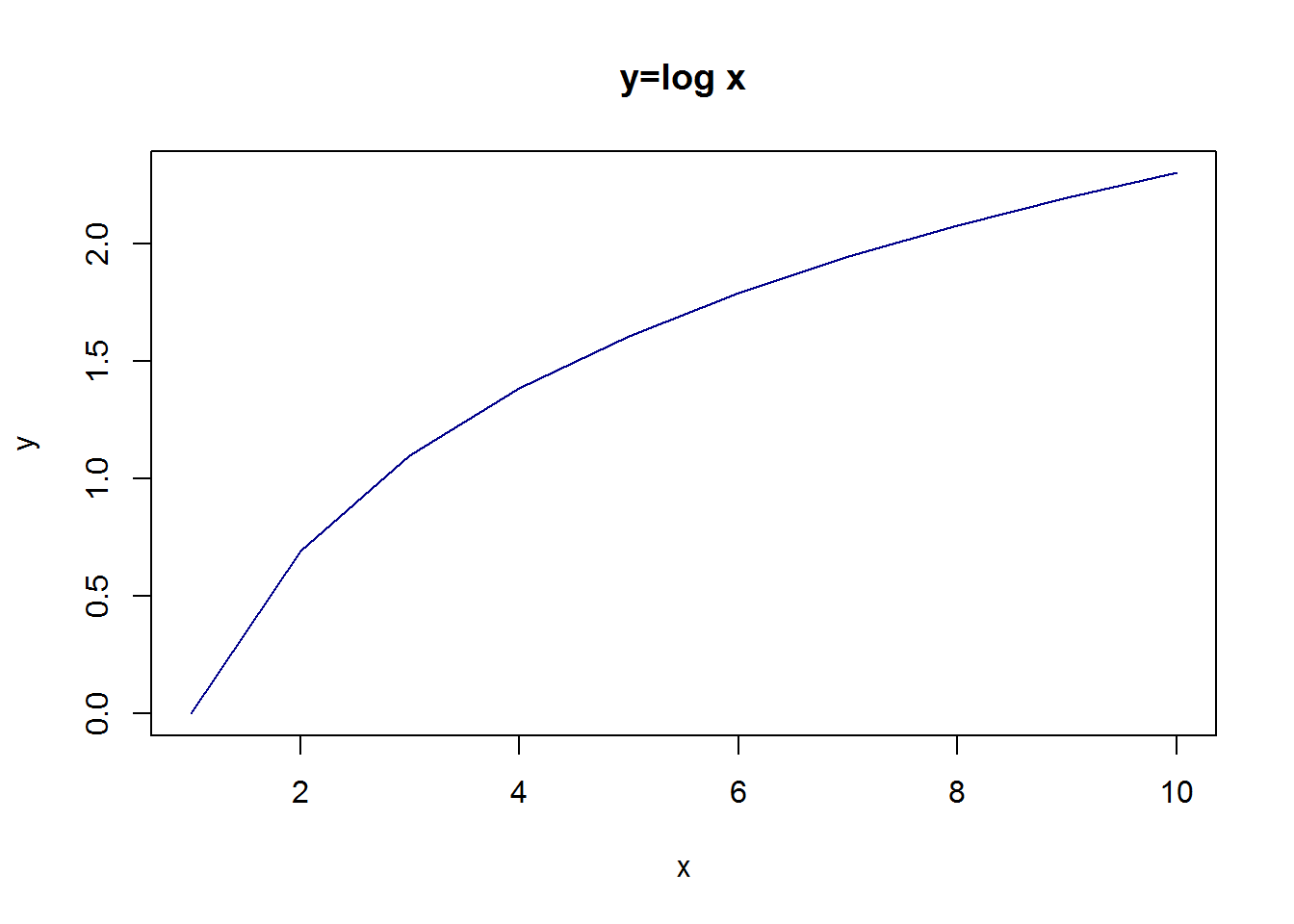### Why Analyze Relationship between 2 Predictor Variables

• It is important to understand the correlations between each pair of predictor variables. Correlated variables lead to multi-collinearity. Essentially, two correlated variables are transmitting the same information, and hence are redundant. Multi-collinearity leads to inflated error term and wider confidence interval (reflecting greater uncertainty in the estimate).
• When there are too many variables in a data-set, we use techniques like PCA for dimensionality reduction. Dimensionality reduction techniques work upon reducing the correlated variables, to reduce the extraneous information and so that we run our modelling algorithms on the variables that explain the maximum variance.

### Method to do Bi-Variate Analysis

We have understood why bi-variate analysis is an essential step to data exploration. Now we will discuss the techniques to bi-variate analysis.

For illustration, we will use Hitters data-set from library ISLR. This is Major League Baseball Data from the 1986 and 1987 seasons. It is a data frame with 322 observations of major league players on 20 variables. The target variable is Salary while the rest of the 19 are dependent variables. Through this data-set, we will demonstrate bi-variate analysis between:

• two continuous variables,
• one continuous and one categorical variable,
• two categorical variables

## Techniques to do Bi-Variate Analysis.

Please note, that the way to do the bi-variate analysis is same irrespective of predictor or target variable.

### Bi-Variate Analysis between Two Continuous Variables

To do the bi-variate analysis between two continuous variables, we have to look at scatter plot between the two variables. The pattern of the scatter plot indicates the relationship between the two variables. As long as there is a pattern between two variables, there can be a transformation applied to the predictor / target variable to achieve a linear relationship for modelling purpose. If no pattern is observed, this implies no relationship possible between the two variables. The strength of the linear relationship between two continuous variables can be quantified using Pearson Correlation.A correlation coefficient of -1 indicates high negative correlation, 0 indicates no correlation, 1 indicates high positive correlation.

Correlation is simply the normalized co-variance with the standard deviation of both the factors. This is done to ensure we get a number between +1 and -1. Co-variance is very difficult to compare as it depends on the units of the two variables. So, we prefer to use correlation for the same.

Please note: * If two variables are linearly related, it means they should have high Pearson Correlation Coefficient. * If two variables are correlated does not indicate they are linearly related. This is because correlation is deeply impacted by outliers. Eg. the correlation for both the below graphs is same, but the linear relation is not there in the second graph: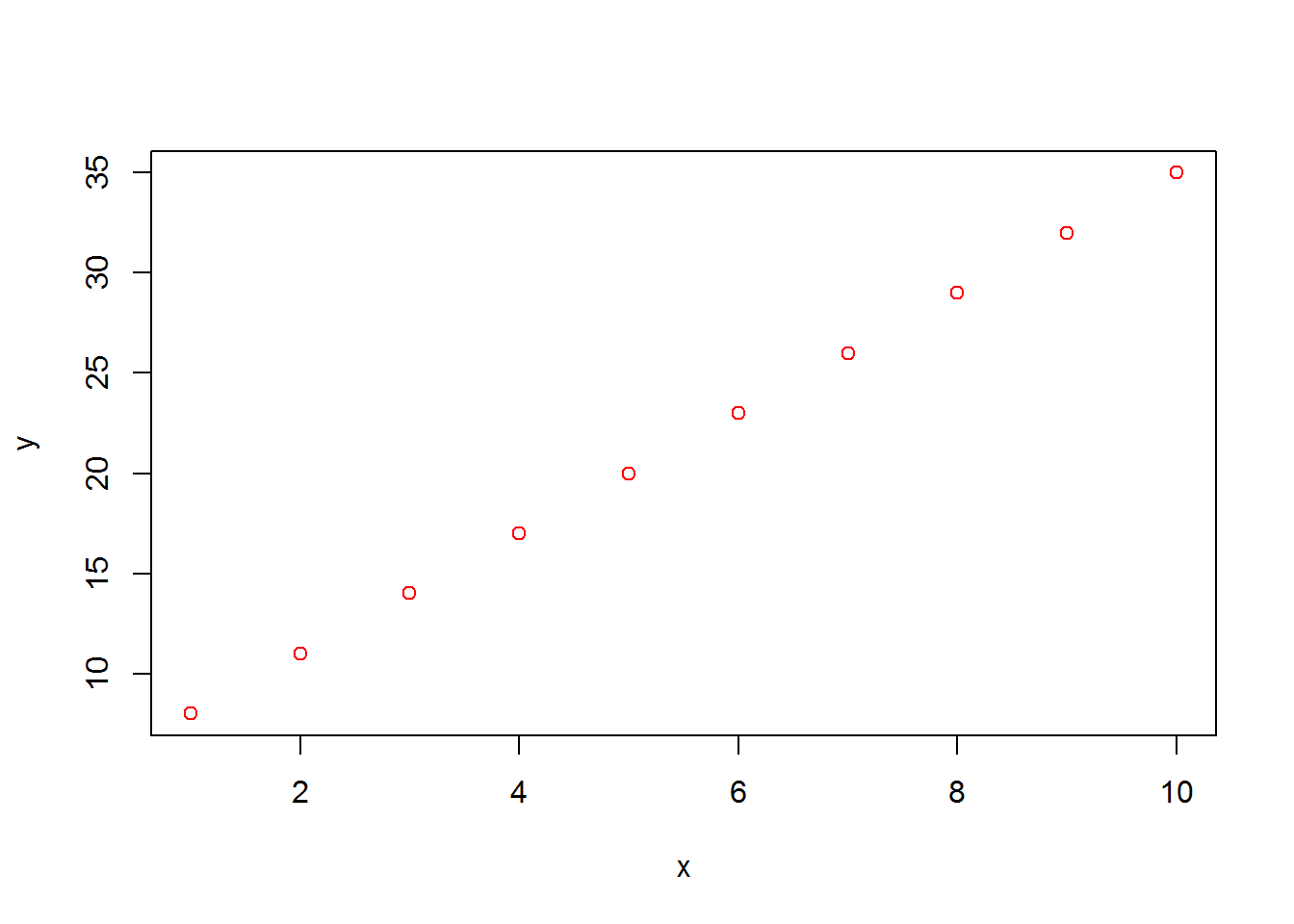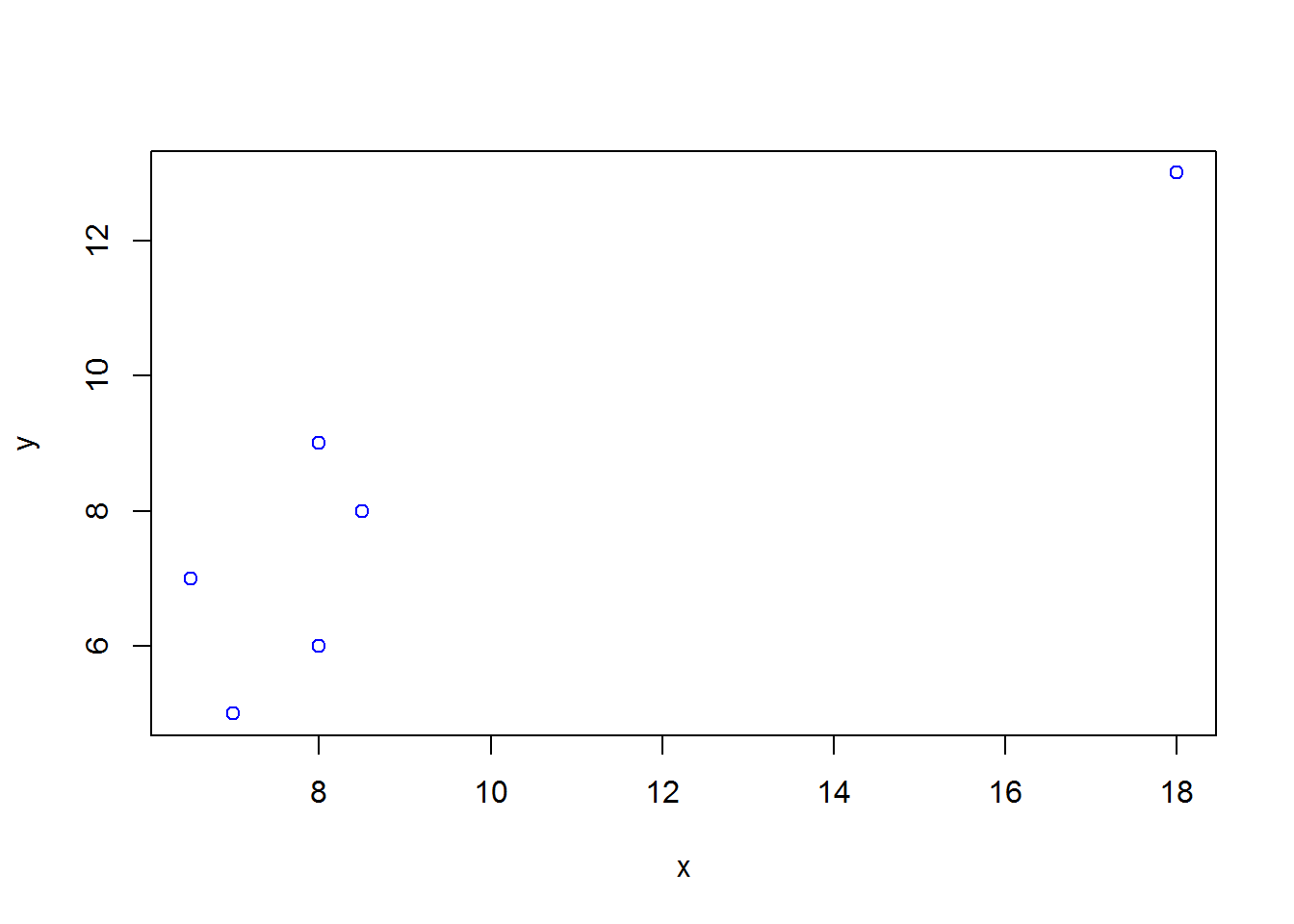• If two variables are related, it does not mean they are necessarily correlated. Eg. in the below graph of y=x^2-1, x and y are related but not correlated (with correlation coefficient of 0).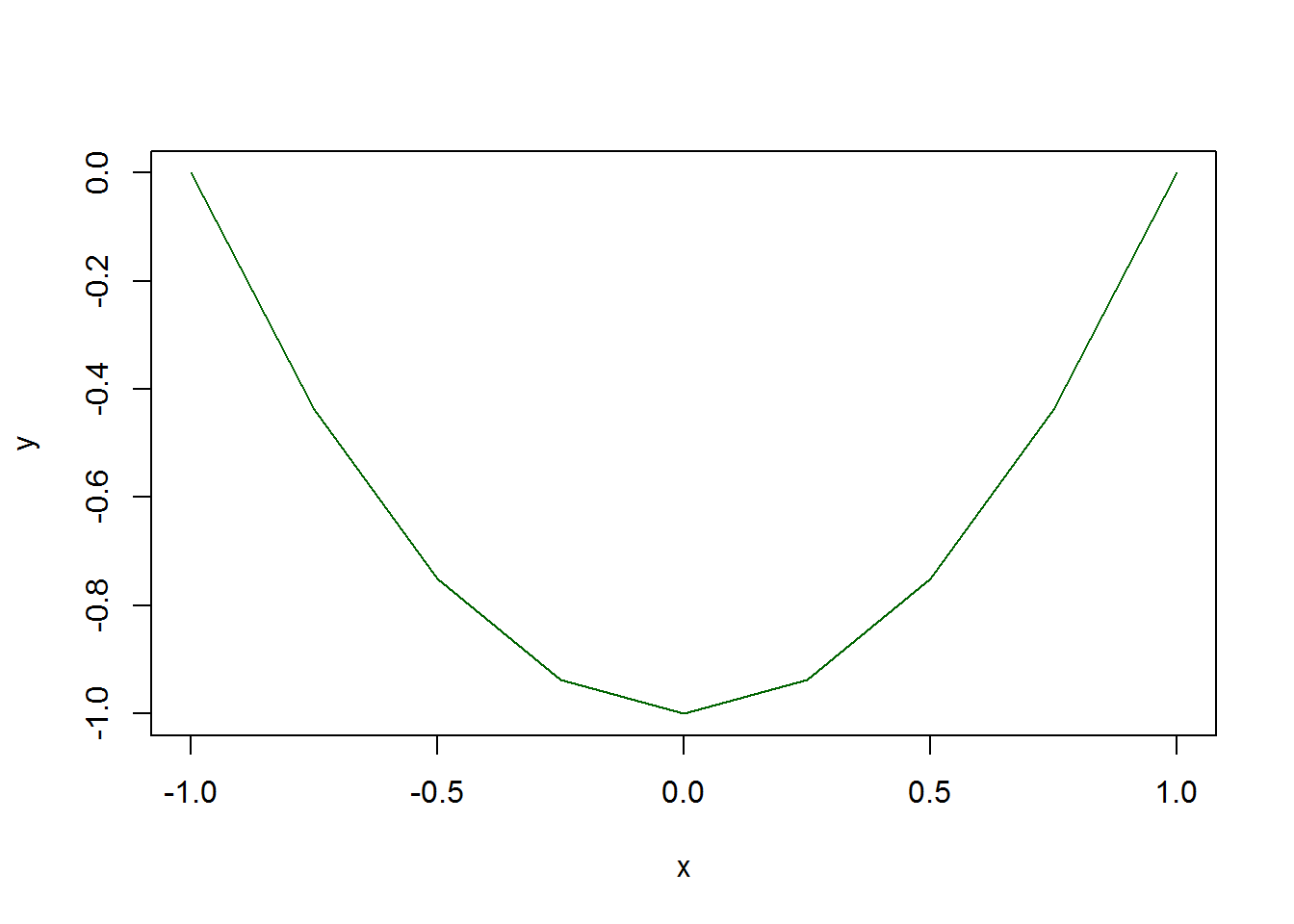#### For our hitters illustration, following are the correlations and the scatter-plots:

This gives the correlation-coefficients between the continuous variables in hitters data-set. Since it is difficult to analyse so many values, we prefer to attain a quick visualization of correlation through scatter plots. Following command gives the scatter plots for the first 4 continuous variables in hitters data-set: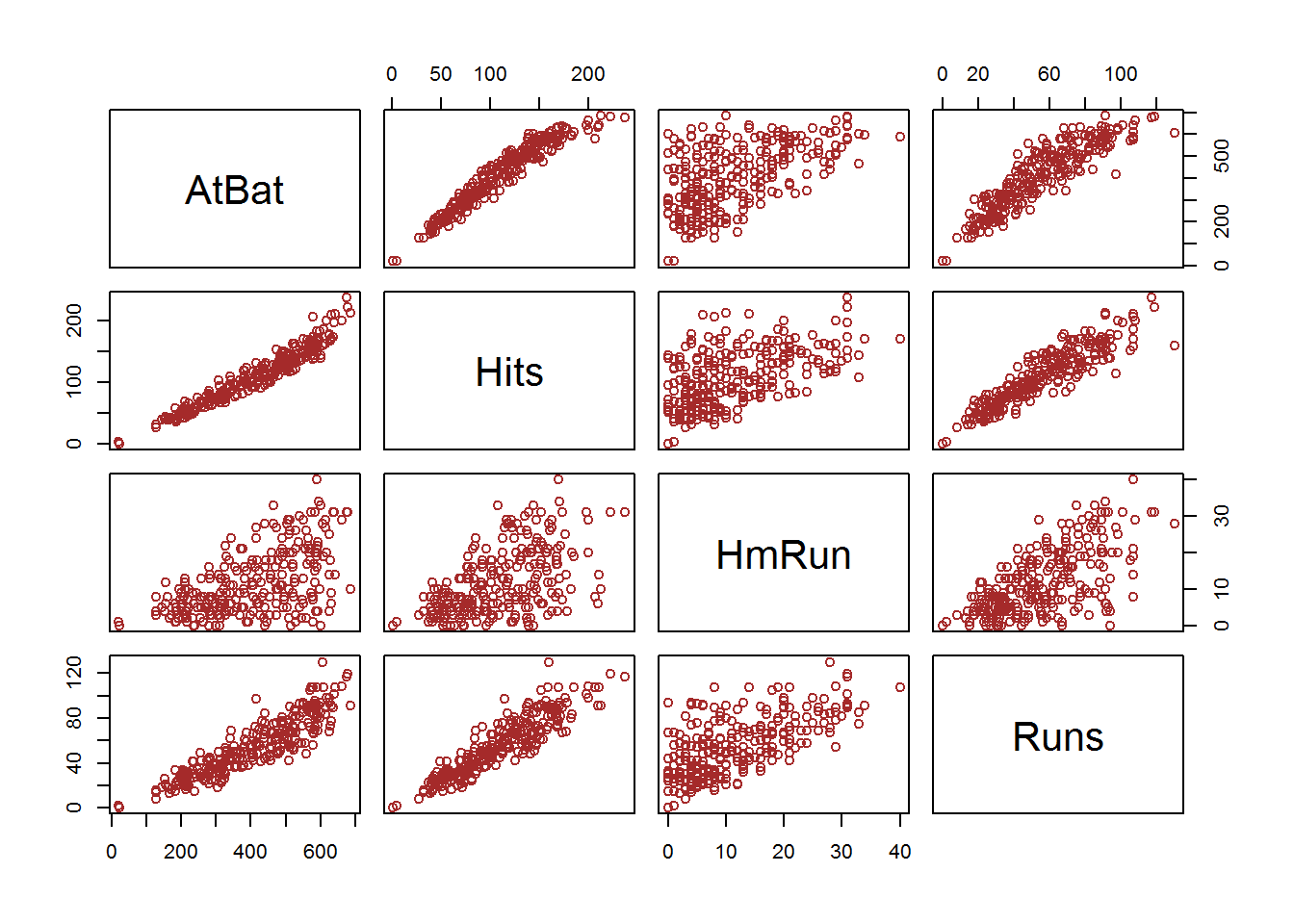#### Observations:

• Linear pattern can be observed between AtBat and Hits, and can be confirmed from the correlation value = 0.96
• Linear pattern can be observed between Hits and Runs, and can be confirmed from the correlation value = 0.91
• Linear pattern can be observed between AtBat and Runs, and can be confirmed from the correlation value = .899

#### To get a scatter plot and correlation between two continuous variables: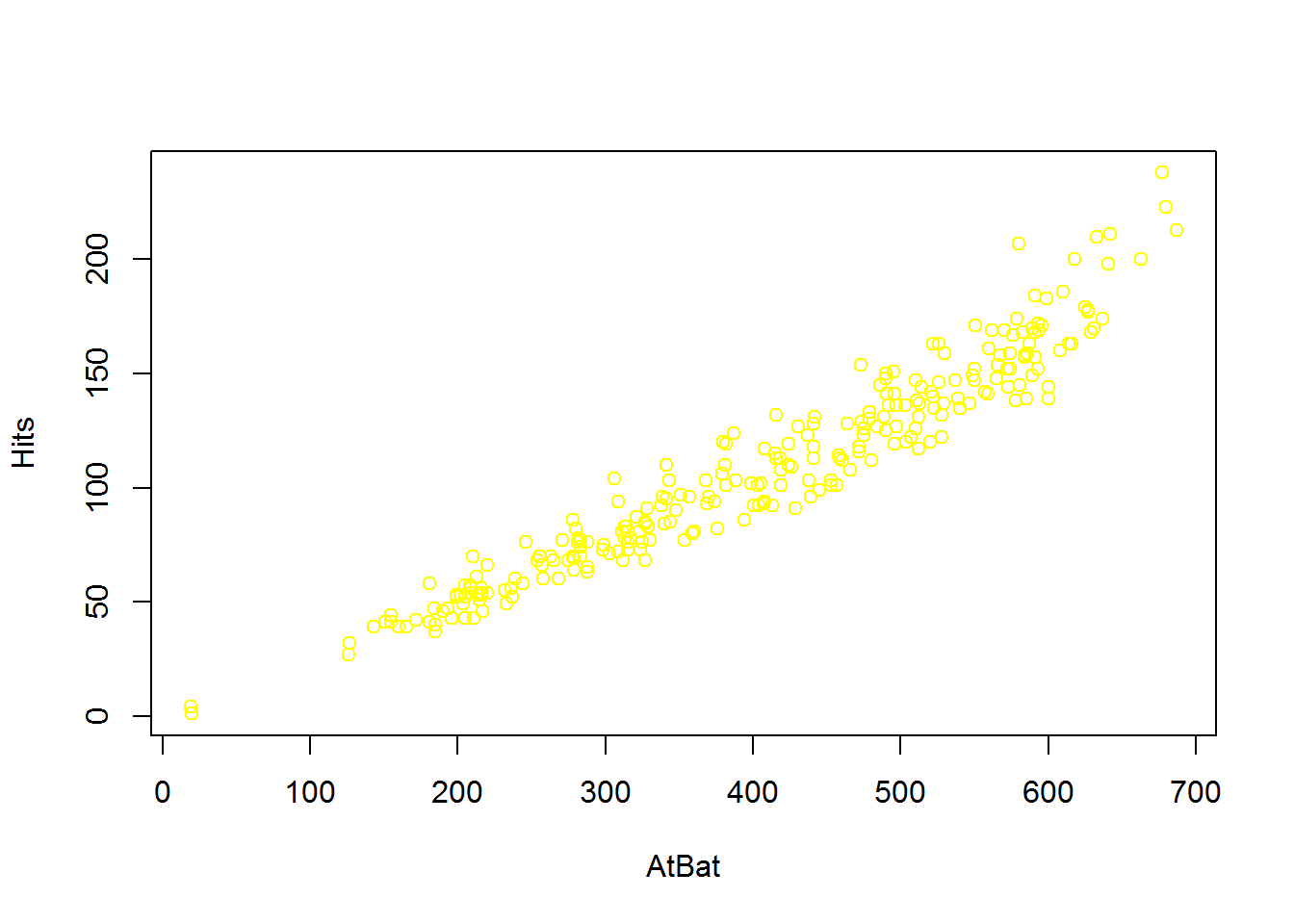The graph shows a strong positive linear correlation.

#### Pearson Correlation Coefficient between AtBat and Hits

Correlation value of .96 verifies our claim of strong positive correlation. We can obtain better visualizations of the correlations through library corrgram and corrplot: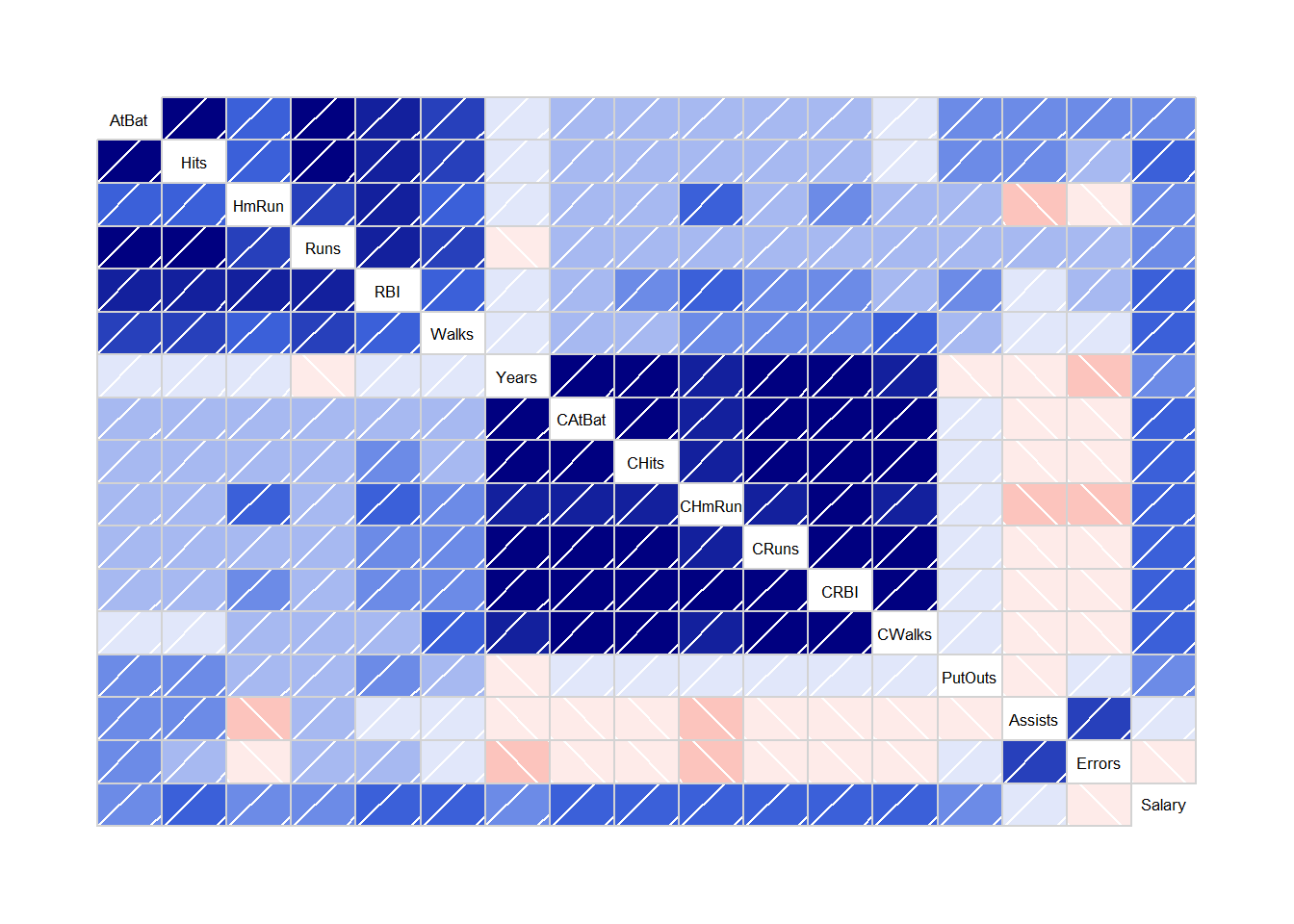Strong blue means strong +ve correlation. Strong red means strong negative correlation. Dark color means strong correlation. Weak color means weak correlation.